# Including fixed effects of covariates

#### 2022-03-23

In the tutorial about how to handle replication units, we learned how to incorporate replicates in a network model. However, the parameter values estimated by the model were shared across all replicates.

In this tutorial, we’ll learn how we can use replicates to estimate the fixed effect of some covariates on the model parameters when the covariates vary across replicates.

We will use a simulated dataset quite similar to the one from the replication tutorial. The modelled foodweb has three compartments:

• dissolved ammonium NH$$_4^+$$ (NH4), which is enriched in $$^{15}$$N at the beginning of the experiment
• planctonic algae which incorporate NH$$_4^+$$
• Daphnia which graze on algae and excrete ammonium into the water.

The experiment is done in two aquariums as before, but this time one aquarium is exposed to light while the other is kept in the dark. How does this treatment affect nitrogen flow? Note that in a real life experiment, we would need more than one replicate per level of the light treatment (otherwise we could not differentiate between treatment effect and replicate effect) - the example in this tutorial is kept excessively simple to focus on the package interface to specify fixed effects.

library(isotracer)
library(tidyverse)

## Data preparation

The simulated data we use in this example can be loaded into your R session by running the code below:

exp <- tibble::tribble(
~time.day,  ~species, ~biomass, ~prop15N, ~treatment,
0,     "NH4",    0.205,    0.739,    "light",
2,     "NH4",    0.232,    0.403,    "light",
4,     "NH4",       NA,    0.199,    "light",
6,     "NH4",       NA,    0.136,    "light",
8,     "NH4",    0.306,       NA,    "light",
10,     "NH4",    0.323,   0.0506,    "light",
0,   "algae",    0.869,  0.00305,    "light",
2,   "algae",       NA,   0.0875,    "light",
4,   "algae",     0.83,    0.131,    "light",
6,   "algae",    0.706,       NA,    "light",
10,   "algae",    0.666,   0.0991,    "light",
0, "daphnia",     2.13,  0.00415,    "light",
2, "daphnia",     1.99,       NA,    "light",
4, "daphnia",     1.97,   0.0122,    "light",
6, "daphnia",       NA,   0.0284,    "light",
8, "daphnia",       NA,   0.0439,    "light",
10, "daphnia",      1.9,   0.0368,    "light",
0,     "NH4",    0.474,     0.98,     "dark",
2,     "NH4",    0.455,     0.67,     "dark",
4,     "NH4",    0.595,    0.405,     "dark",
6,     "NH4",       NA,    0.422,     "dark",
10,     "NH4",    0.682,    0.252,     "dark",
0,   "algae",     1.06,  0.00455,     "dark",
2,   "algae",        1,   0.0637,     "dark",
4,   "algae",    0.862,   0.0964,     "dark",
6,   "algae",       NA,    0.222,     "dark",
8,   "algae",       NA,    0.171,     "dark",
10,   "algae",    0.705,    0.182,     "dark",
0, "daphnia",     1.19,  0.00315,     "dark",
4, "daphnia",     1.73,   0.0204,     "dark",
6, "daphnia",     1.75,       NA,     "dark",
8, "daphnia",     1.54,   0.0598,     "dark",
10, "daphnia",     1.65,   0.0824,     "dark"
)

As shown in the dataset, the first aquarium is exposed to light and the second one is kept in the dark. We trace the nitrogen fluxes by adding $$^{15}$$N-enriched ammonium at the beginning of the experiment. Let’s visualize the data:

library(ggplot2)
library(gridExtra)
p1 <- ggplot(exp, aes(x = time.day, y = biomass, col = species)) +
geom_point() + ggtitle("Biomass data") + ylab("Biomass (mg N)") +
facet_wrap(~ treatment)
p2 <- ggplot(exp, aes(x = time.day, y = prop15N, col = species)) +
geom_point() + ggtitle("Heavy isotope proportions") + ylab("Proportion of 15N")  +
facet_wrap(~ treatment)
grid.arrange(p1, p2, nrow = 2)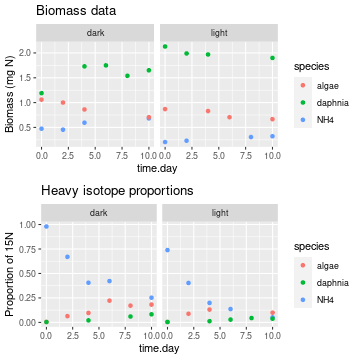## Building the model

We separate the initial conditions and the observations:

inits <- exp %>% filter(time.day == 0)
obs <- exp %>% filter(time.day > 0)

We build the network model, using treatment as a grouping variable:

m <- new_networkModel() %>%
set_topo("NH4 -> algae -> daphnia -> NH4") %>%
set_init(inits, comp = "species", size = "biomass", prop = "prop15N",
group_by = "treatment") %>%
set_obs(obs, time = "time.day")
m
## # A tibble: 2 × 5
##   topology           initial          observations      parameters       group
##   <list>             <list>           <list>            <list>           <list>
## 1 <topology [3 × 3]> <tibble [3 × 3]> <tibble [14 × 4]> <tibble [8 × 2]> <chr >
## 2 <topology [3 × 3]> <tibble [3 × 3]> <tibble [13 × 4]> <tibble [8 × 2]> <chr >

The network model object m has two rows, corresponding to the two treatments:

groups(m)
## # A tibble: 2 × 1
##   treatment
##   <chr>
## 1 light
## 2 dark

If we go on and run the MCMC now, the two treatments will share the same parameter values and will only act as simple replicates, without any covariate effect. We need to specify that the treatment grouping variable is to be used as a covariate for some of the parameters estimated by the model.

## Specifying fixed effect covariates

We specify covariates with the add_covariates() function and the formula syntax parameters ~ covariates where parameters is the list of parameters affected by one or several covariates specified in covariates.

For example, to tell the model that the nitrogren flux from NH$$_4^+$$ to algae should depend on the treatment, we type:

m <- m %>% add_covariates(upsilon_NH4_to_algae ~ treatment)

Let’s have a look at the model parameters at this stage:

params(m)
## # A tibble: 9 × 2
##   in_model                   value
##   <chr>                      <dbl>
## 1 eta                           NA
## 2 lambda_algae                  NA
## 3 lambda_daphnia                NA
## 4 lambda_NH4                    NA
## 5 upsilon_algae_to_daphnia      NA
## 6 upsilon_daphnia_to_NH4        NA
## 7 upsilon_NH4_to_algae|dark     NA
## 8 upsilon_NH4_to_algae|light    NA
## 9 zeta                          NA

We can see that there are now two entries for upsilon_NH4_to_algae: upsilon_NH4_to_algae|light and upsilon_NH4_to_algae|dark. All the other parameters are unaffected by the treatment covariate.

We can specify covariate specifications sequentially. For example, we can now tell the model that the nitrogen flux from algae to Daphnia also depends on the treatment:

m <- m %>% add_covariates(upsilon_algae_to_daphnia ~ treatment)

and we can have a detailed look at the current covariate specification by looking at the parameter mapping in each replicate:

m$parameters ## [] ## # A tibble: 8 × 2 ## in_replicate in_model ## <chr> <chr> ## 1 eta eta ## 2 lambda_algae lambda_algae ## 3 lambda_daphnia lambda_daphnia ## 4 lambda_NH4 lambda_NH4 ## 5 upsilon_algae_to_daphnia upsilon_algae_to_daphnia|light ## 6 upsilon_daphnia_to_NH4 upsilon_daphnia_to_NH4 ## 7 upsilon_NH4_to_algae upsilon_NH4_to_algae|light ## 8 zeta zeta ## ## [] ## # A tibble: 8 × 2 ## in_replicate in_model ## <chr> <chr> ## 1 eta eta ## 2 lambda_algae lambda_algae ## 3 lambda_daphnia lambda_daphnia ## 4 lambda_NH4 lambda_NH4 ## 5 upsilon_algae_to_daphnia upsilon_algae_to_daphnia|dark ## 6 upsilon_daphnia_to_NH4 upsilon_daphnia_to_NH4 ## 7 upsilon_NH4_to_algae upsilon_NH4_to_algae|dark ## 8 zeta zeta Here, we can see that upsilon_NH4_to_algae and upsilon_algae_to_daphnia within each replicate depend on light and dark, while all the other parameters are shared across replicates. The formula syntax in add_covariates() is quite versatile and can perform partial matching. For example, if we want all the loss rates to depend on the treatment, we can use: m <- m %>% add_covariates(lambda ~ treatment) and all the parameters containing the string lambda will be affected in one go: m$parameters
## []
## # A tibble: 8 × 2
##   in_replicate             in_model
##   <chr>                    <chr>
## 1 eta                      eta
## 2 lambda_algae             lambda_algae|light
## 3 lambda_daphnia           lambda_daphnia|light
## 4 lambda_NH4               lambda_NH4|light
## 5 upsilon_algae_to_daphnia upsilon_algae_to_daphnia|light
## 6 upsilon_daphnia_to_NH4   upsilon_daphnia_to_NH4
## 7 upsilon_NH4_to_algae     upsilon_NH4_to_algae|light
## 8 zeta                     zeta
##
## []
## # A tibble: 8 × 2
##   in_replicate             in_model
##   <chr>                    <chr>
## 1 eta                      eta
## 2 lambda_algae             lambda_algae|dark
## 3 lambda_daphnia           lambda_daphnia|dark
## 4 lambda_NH4               lambda_NH4|dark
## 5 upsilon_algae_to_daphnia upsilon_algae_to_daphnia|dark
## 6 upsilon_daphnia_to_NH4   upsilon_daphnia_to_NH4
## 7 upsilon_NH4_to_algae     upsilon_NH4_to_algae|dark
## 8 zeta                     zeta

To affect all parameters, one can use . on the left-hand side of the formula:

m <- m %>% add_covariates(. ~ treatment)

Finally, to specify that a parameter does not depend on any covariate and is shared across replicates, one can use 1 on the right-hand side of the formula:

m <- m %>% add_covariates(zeta ~ 1)

which means that we can remove all fixed effects for all parameters with:

m <- m %>% add_covariates(. ~ 1)

For this tutorial, let’s assume that all nitrogen fluxes across compartments can depend on the light treatment. The parameters corresponding to those fluxes are the ones starting with upsilon:

m <- m %>% add_covariates(upsilon ~ treatment)
params(m)
## # A tibble: 11 × 2
##    in_model                       value
##    <chr>                          <dbl>
##  1 eta                               NA
##  2 lambda_algae                      NA
##  3 lambda_daphnia                    NA
##  4 lambda_NH4                        NA
##  5 upsilon_algae_to_daphnia|dark     NA
##  6 upsilon_algae_to_daphnia|light    NA
##  7 upsilon_daphnia_to_NH4|dark       NA
##  8 upsilon_daphnia_to_NH4|light      NA
##  9 upsilon_NH4_to_algae|dark         NA
## 10 upsilon_NH4_to_algae|light        NA
## 11 zeta                              NA

## Running the MCMC

We quickly set some reasonable vague priors for the particular model at hand:

m <- set_priors(m, normal_p(0, 4), "")
priors(m)
## # A tibble: 11 × 2
##    in_model                       prior
##    <chr>                          <list>
##  1 eta                            <trun_normal(mean=0,sd=4)>
##  2 lambda_algae                   <trun_normal(mean=0,sd=4)>
##  3 lambda_daphnia                 <trun_normal(mean=0,sd=4)>
##  4 lambda_NH4                     <trun_normal(mean=0,sd=4)>
##  5 upsilon_algae_to_daphnia|dark  <trun_normal(mean=0,sd=4)>
##  6 upsilon_algae_to_daphnia|light <trun_normal(mean=0,sd=4)>
##  7 upsilon_daphnia_to_NH4|dark    <trun_normal(mean=0,sd=4)>
##  8 upsilon_daphnia_to_NH4|light   <trun_normal(mean=0,sd=4)>
##  9 upsilon_NH4_to_algae|dark      <trun_normal(mean=0,sd=4)>
## 10 upsilon_NH4_to_algae|light     <trun_normal(mean=0,sd=4)>
## 11 zeta                           <trun_normal(mean=0,sd=4)>

We run the MCMC as usual:

run <- run_mcmc(m, iter = 1000)
plot(run)
# Note: the figure below only shows a few of the traceplots for vignette concision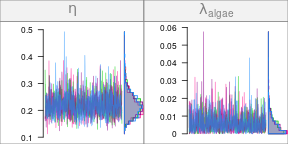and we do a posterior predictive check:

predictions <- predict(m, run)
plot(predictions, facet_row = c("group", "type"),
facet_col = "compartment",
scale = "all")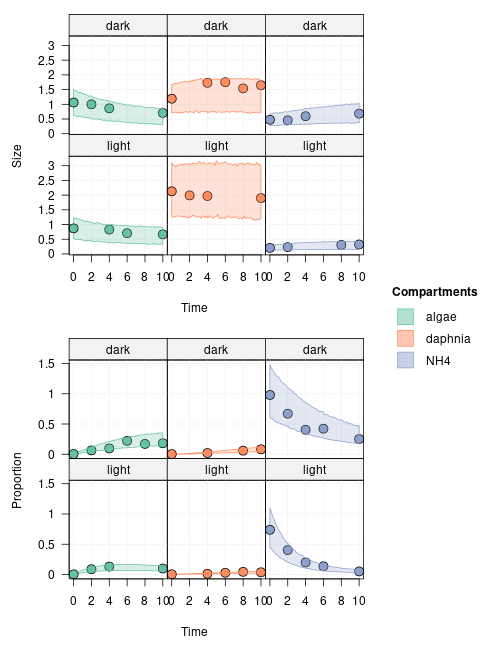## Interpreting the output

Let’s see if the upsilon parameters were actually different between the light and dark treatments:

summary(run %>% select(upsilon))
##
## Iterations = 501:1000
## Thinning interval = 1
## Number of chains = 4
## Sample size per chain = 500
##
## 1. Empirical mean and standard deviation for each variable,
##    plus standard error of the mean:
##
##                                   Mean       SD  Naive SE Time-series SE
## upsilon_algae_to_daphnia|dark  0.12328 0.015693 0.0003509      0.0003922
## upsilon_algae_to_daphnia|light 0.13958 0.018661 0.0004173      0.0004367
## upsilon_daphnia_to_NH4|dark    0.05692 0.009839 0.0002200      0.0002592
## upsilon_daphnia_to_NH4|light   0.04074 0.004722 0.0001056      0.0001146
## upsilon_NH4_to_algae|dark      0.08598 0.011618 0.0002598      0.0002647
## upsilon_NH4_to_algae|light     0.28468 0.026662 0.0005962      0.0006695
##
## 2. Quantiles for each variable:
##
##                                   2.5%     25%     50%     75%   97.5%
## upsilon_algae_to_daphnia|dark  0.09480 0.11312 0.12298 0.13310 0.15584
## upsilon_algae_to_daphnia|light 0.10498 0.12708 0.13847 0.15134 0.18103
## upsilon_daphnia_to_NH4|dark    0.04083 0.05012 0.05598 0.06235 0.07990
## upsilon_daphnia_to_NH4|light   0.03245 0.03748 0.04050 0.04362 0.05063
## upsilon_NH4_to_algae|dark      0.06664 0.07806 0.08494 0.09239 0.11307
## upsilon_NH4_to_algae|light     0.23688 0.26636 0.28385 0.30099 0.33951

Looking at a table of numbers is not the easiest way to visualize the differences between parameter values. One could take advantage of the bayesplot package for a more visual output:

library(bayesplot)
mcmc_intervals(run %>% select(upsilon)) +
coord_trans(x = "log10")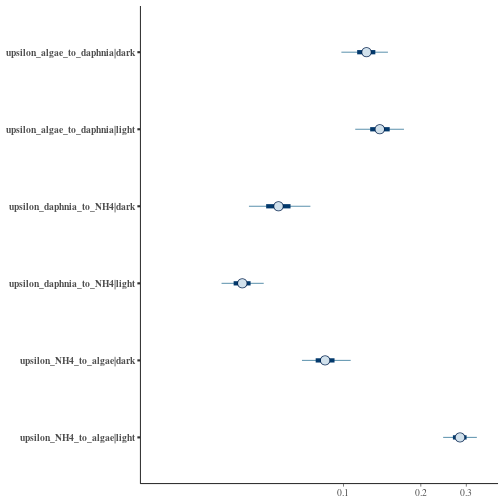Based on this plot, it looks like only the rates from ammonium to algae (upsilon_NH4_to_algae) actually differ between the light and the dark treatments.

Let’s check this more rigorously. One nice thing about Bayesian MCMC is that we can combine the traces of primary parameters sampled during the MCMC to generate posteriors for derived parameters. We want to see the posterior for the ratio between the uptake rate coefficients for NH4 -> algae in the light and in the dark treatments:

ratio_upsilons_NH4_algae <- (run[, "upsilon_NH4_to_algae|light"] /
run[, "upsilon_NH4_to_algae|dark"])
plot(ratio_upsilons_NH4_algae)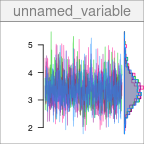As we can see above, the posterior for the ratio $$\frac{\upsilon_{NH4 \rightarrow algae}|light}{\upsilon_{NH4 \rightarrow algae}|dark}$$ is far from one. We can check that with the numerical summary:

summary(ratio_upsilons_NH4_algae)
##
## Iterations = 501:1000
## Thinning interval = 1
## Number of chains = 4
## Sample size per chain = 500
##
## 1. Empirical mean and standard deviation for each variable,
##    plus standard error of the mean:
##
##           Mean             SD       Naive SE Time-series SE
##        3.36850        0.53814        0.01203        0.01347
##
## 2. Quantiles for each variable:
##
##  2.5%   25%   50%   75% 97.5%
## 2.389 3.001 3.343 3.694 4.541

The model tells us that the algae uptake ammonium more rapidly in the light treatment. What about the nitrogen flows between algae and Daphnia? Are the uptake rate coefficients estimated in the light and the dark treatments different?

ratio_upsilons_algae_daphnia <- (run[, "upsilon_algae_to_daphnia|light"] /
run[, "upsilon_algae_to_daphnia|dark"])
plot(ratio_upsilons_algae_daphnia)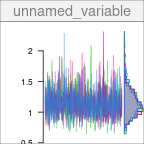For this comparison, the posterior of the ratio overlaps one quite generously: the model does not support an effect of the light/dark treatment on the nitrogen flux between algae and Daphnia.